# Topic Modelling using Unsupervised Learning: LDA and LSA

### Introduction

The expansion of the internet and the easy accessibility of smartphones, with internet access, has led to a boom in the content available for surfing. Although there are a lot of positives from this technological shift, at the same time, uncovering the relevant information has become a pain point. This is where topic modelling shines.

Consider a case of 30 news articles where 5 focus on cricket, 4 on football, 3 on hockey, and the remaining articles focus on laptops and mobiles. Topic modelling helps classify the articles focusing on cricket, football and hockey under sports and the remaining under technology.

In this blog, we explore and compare two techniques for topic modelling: Latent Dirichlet Allocation (LDA) and Latent Semantic Analysis (LSA).

### Key takeaways

• Problem Statement
• Functioning of LDA and LSA
• Dataset Information
• Exploratory Data Analysis
• Building LDA and LSA models

### Problem statement

Events happen daily, most of which are reported by various news agencies and the general public. These reports include the Ukraine war, financial crisis, elections, ecological disasters, etc. The amount of data added daily regarding such events is alarming, and it's almost impossible to classify these events into different topics manually. That's where topic modelling comes into the picture.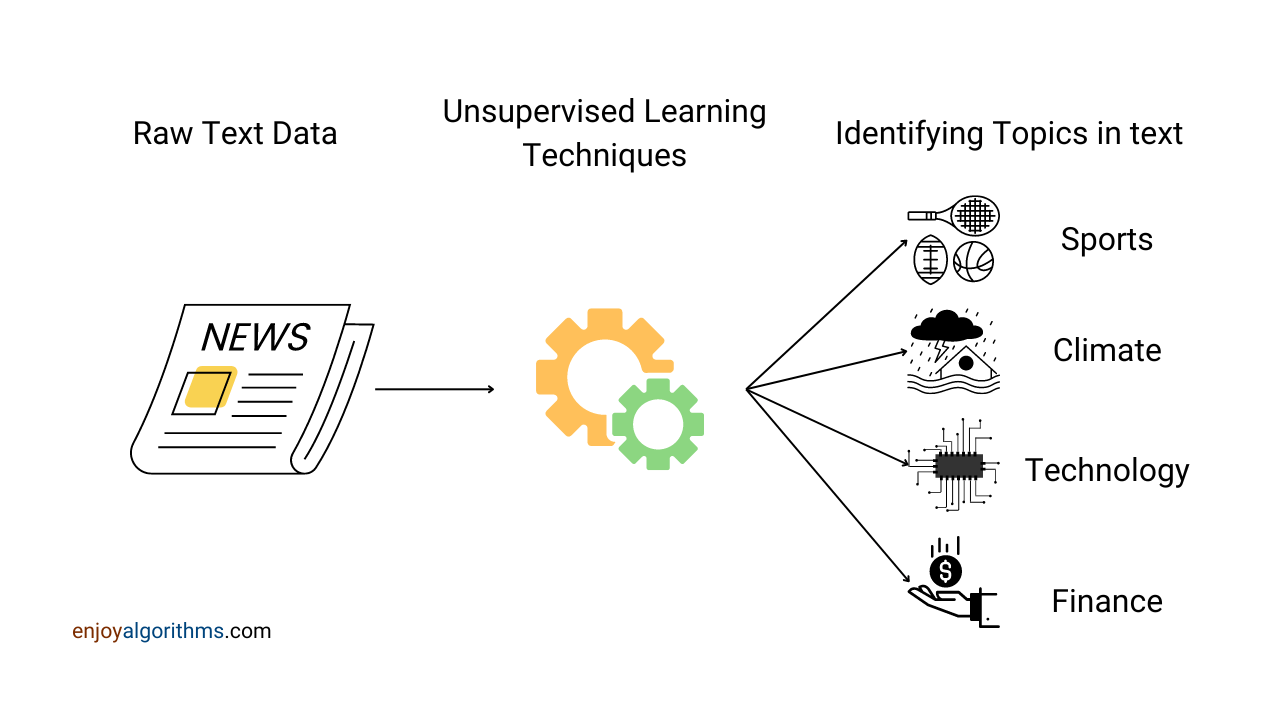Topic modelling is a type of statistical modelling that clubs together similar textual content. It is currently a hot topic in the field of NLP and has a great demand, especially when considering the increasing amount of unstructured data.

In this blog, we will discuss the working of LDA, a basic algorithm used for topic modelling. We execute this algorithm on the 'Million News Headlines' dataset, which is then followed by categorizing the dataset into different topics.

### Functioning of LDA and LSA

LDA and LSA are algorithms that help us to determine topics. Let's learn more about their functioning, but before doing so, let's define a few basic terms to set the context for further discussions.

• Document: A collection of words and instances. It can also be said to be rows of the dataset.
• Body: This represents the entire dataset or a collection of documents.
• Dictionary: The set of all the words that appear at least once anywhere in the body, also known as the vocabulary.
• Topic: A collection of text that represents a similar larger category.
• Latent: Features hidden in the data and cannot be directly measured. In our case, these can be called topics, as these are indirect classifications which can be used to group different documents into classes or topics.

The content available these days is mostly unlabelled, which justifies using unsupervised techniques. LDA and LSA are unsupervised learning methods, making them suitable for the task.

Please note that the number of topics is a required parameter in both algorithms. It can be defined as the number of categories into which the content is expected to be classified. In this blog, we choose the total number of topics to be 10.

#### Latent Dirichlet Allocation (LDA)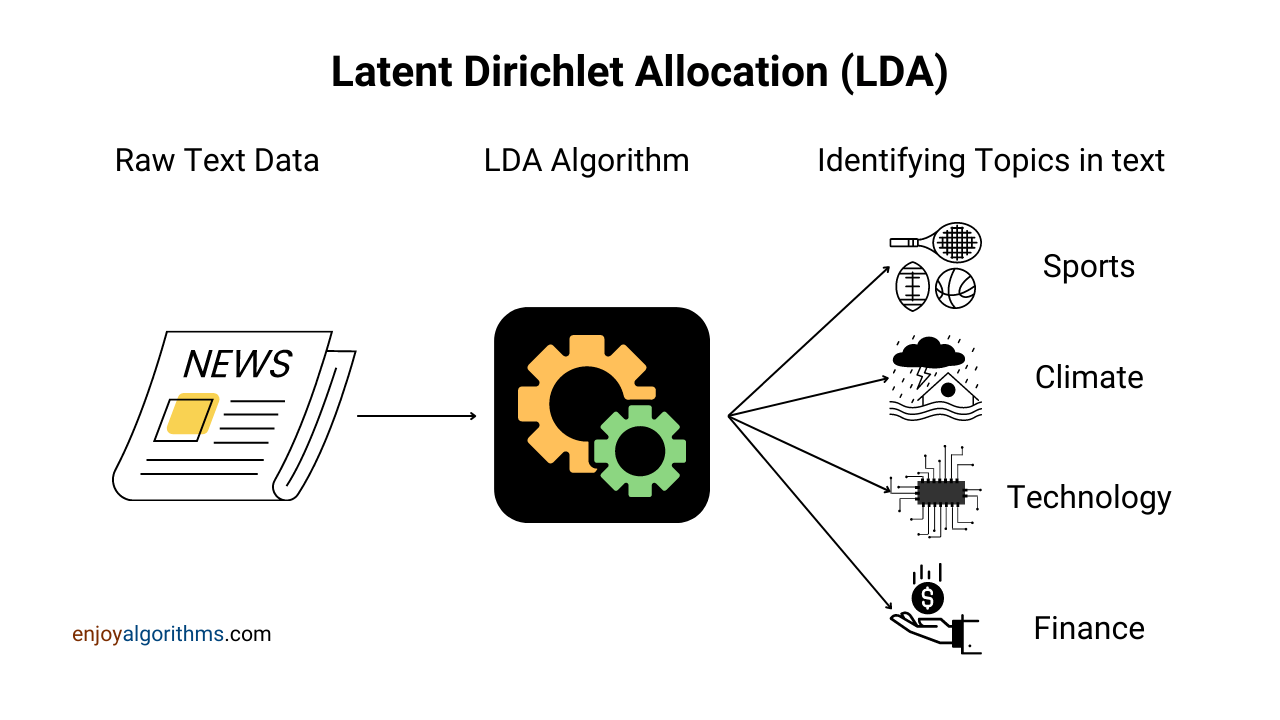LDA algorithm exploits the 'word frequency' in documents to generate topics and is represented as the black box in the above diagram. Let's use an example and set specific assumptions to understand this black box. Assumptions are:

• Only 4 topics are considered.
• An imaginary body of n documents from which the 'i'th document is shown below.

#### Steps involved in LDA

Doc i: GT won the IPL Cup in 2022.

Step 1: A random topic for each word will be assigned:

``````Word/Token  |  GT  | won  |  the  | IPL  |  cup |  in | 2022 |

Topic       |  1   |  4   |   1   |  2   |   3  |   4 |  1   |``````

Step 2: The count of topics per document is prepared:

``````Topic  |  Topic 1  | Topic 2  |  Topic 3  | Topic 4  |

Count  |    3      |    1     |     1     |     2    |``````

Step 3: Across all the documents, the frequency of every topic for each unique word is calculated.

``````Words  | Topic 1 | Topic 2  |  Topic 3   | Topic 4   |
------------------------------------------------------
GT     |    5    |    3     |     2      |     9     |

won    |    3    |    7     |     4      |     14    |

the    |    6    |    8     |     14     |     18    |

IPL    |    8    |    4     |     2      |     27    |

cup    |    5    |    9     |     19     |     6     |

in     |    10   |    12    |     9      |     7     |

2022   |    13   |    15    |     4      |     2     |``````

Step 4: A random word is picked up, and the topic referred to by the word is reset in all documents sequentially where this word was present. In this example, let's select 'IPL', and it will not have any topic in the 'i'th document. Correspondingly the metric from steps 2 and step 3 will also change.

Step 5: A new topic must be assigned to the word IPL. It is done based on the score of

• metric_A= How much a document likes the topic (Step2 gives us this value)
• metric_B= How many topics like the selected word (for each topic, we get this value from step 3)

Overall score = metricA * metric

The above score is calculated for every topic for document i. The topic with the max score is assigned to the word 'IPL', which is topic 4.
Overall score for topic 4 = 2 * 27

Step 6: These step 4 and step 5 are repeated for every unique word in the body.

Step 7: Step 6 is done a fixed number of times

#### Latent Semantic Analysis (LSA)

LSA is a method which helps us to convert unstructured data to a structured form. The below diagram describes the process of LSA.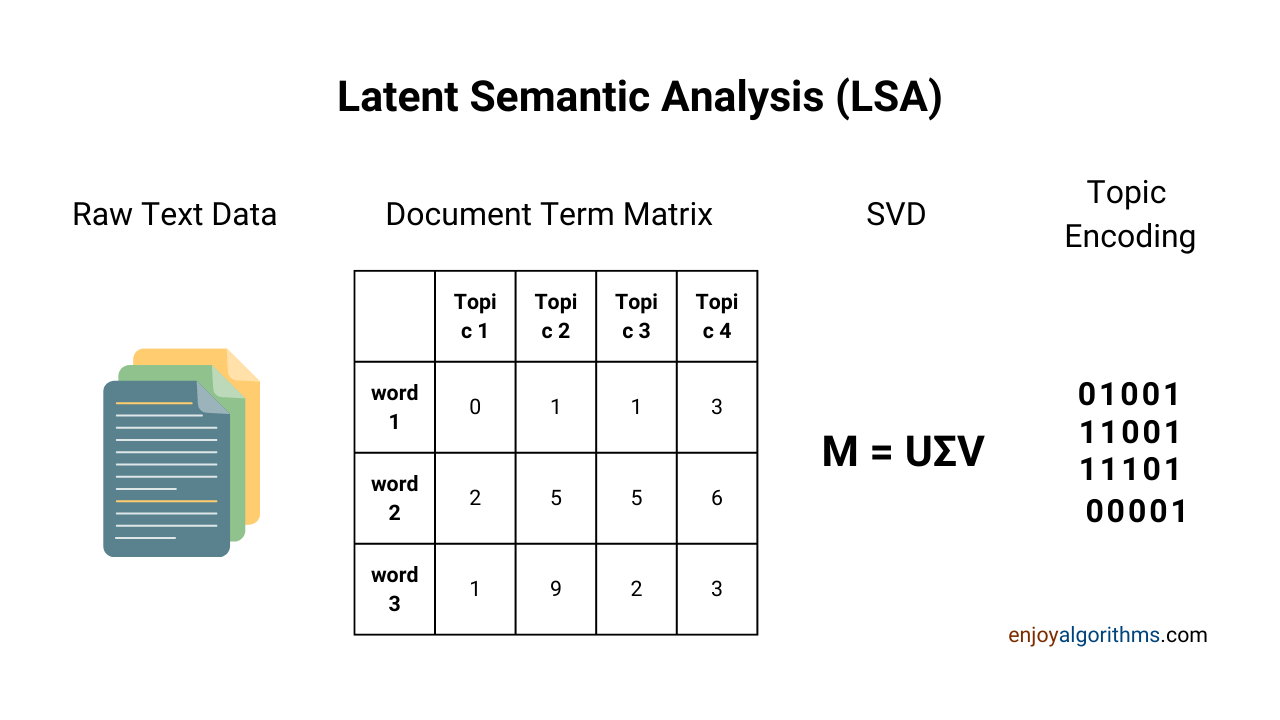Let's look into some of the key components mentioned above →

• Document Term Matrix: Documents are nothing but merely a collection of text, and this text is not helpful until we translate them into a machine-readable format as it can only understand numbers. To represent documents in the form of numerical vectors is known as the document term matrix. This can be created using various techniques, such as count vectorizer, TF-IDF, Word2Vec etc. More details about these techniques can be read in our blog on word-vector encoding.
• Singular Value Decomposition (SVD): It is a matrix factorization method and helps to bring the document term matrix to a lower dimension like PCA. But the underlying principles of SVD are different from PCA. Lowering the dimensions involves forming groups based on latent features, which in this Case are topics.

### Implementing LDA and LSA using Python

The basics of LDA and LSA are covered. Let's start implementing them. The basic steps that will be followed here are:

1. Selection and understanding of the dataset to be used.
2. EDA (Exploratory Data Analysis) to understand the data distribution and gain insights.
3. Model building and performing inference through that on unseen data.
4. Comparison of the developed model with other models to select the better.

### Dataset information

The dataset we choose for this blog is "A Million News Headlines". It contains news headlines of the past nineteen years from the Australian Broadcasting Corporation (ABC), a reputable Australian news source. A sample from the data is shown below.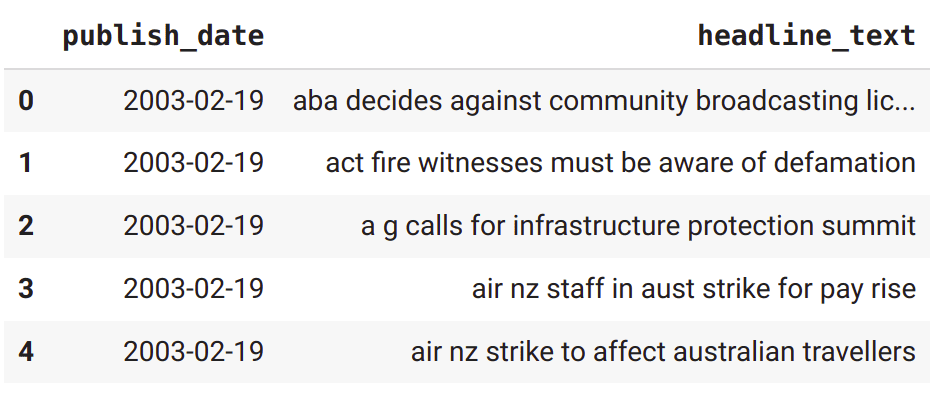This dataset contains the following fields →

• publish_date: This field contains the date when the news was published.
• headline_text: This field includes the headlines picked from the ABC website.

``````import pandas as pd

The site from which we picked the data claims that the dataset contains every news from the ABC website and has witnessed more than 200 news per day. Let's verify this thing through EDA in the next section.

### Pre-processing and Exploratory Data Analysis (EDA)

The dataset should generally be pre-processed before it is used for any downstream tasks. Some of the common pre-processing steps are as follows →

• Removing Stopwords: Stopwords are common words used frequently in any language. Words such as 'the', 'is' etc. are stopwords. Intuitively, removing this makes sense because stop words are present in almost every sentence and do not carry any distinguishing feature about the text, such as the topic being discussed. The Spacy library can be used to get a list of stopwords and help remove them from sentences.
``````import spacy
from spacy.lang.en.stop_words import STOP_WORDS as stopwords
x1 = ' '.join([w for w in x1.split() if w not in stopwords])
## here x1 refers to any news heading``````
• White Spaces: The text might have more than one white space between consecutive words. These should be addressed before feeding it to the downstream tasks so that similar word sequences stay together rather than get separated because of extra white spaces.
• Lower Case: We do not want different cased words to set different contexts, as their meaning remains the same. Hence the whole text is converted to lowercase before feeding it to the downstream tasks.

Pre-processing is now complete. Let's perform an EDA on critical characteristics to understand the data better.

#### Top Words Without Stopwords

If we do not remove the stopwords, the top words mostly consist of stopwords like 'the', 'is ', 'are ', and 'an', as they are most frequently used in any sentence. After their removal, the top words occurring in the body are drastically changed, as shown below.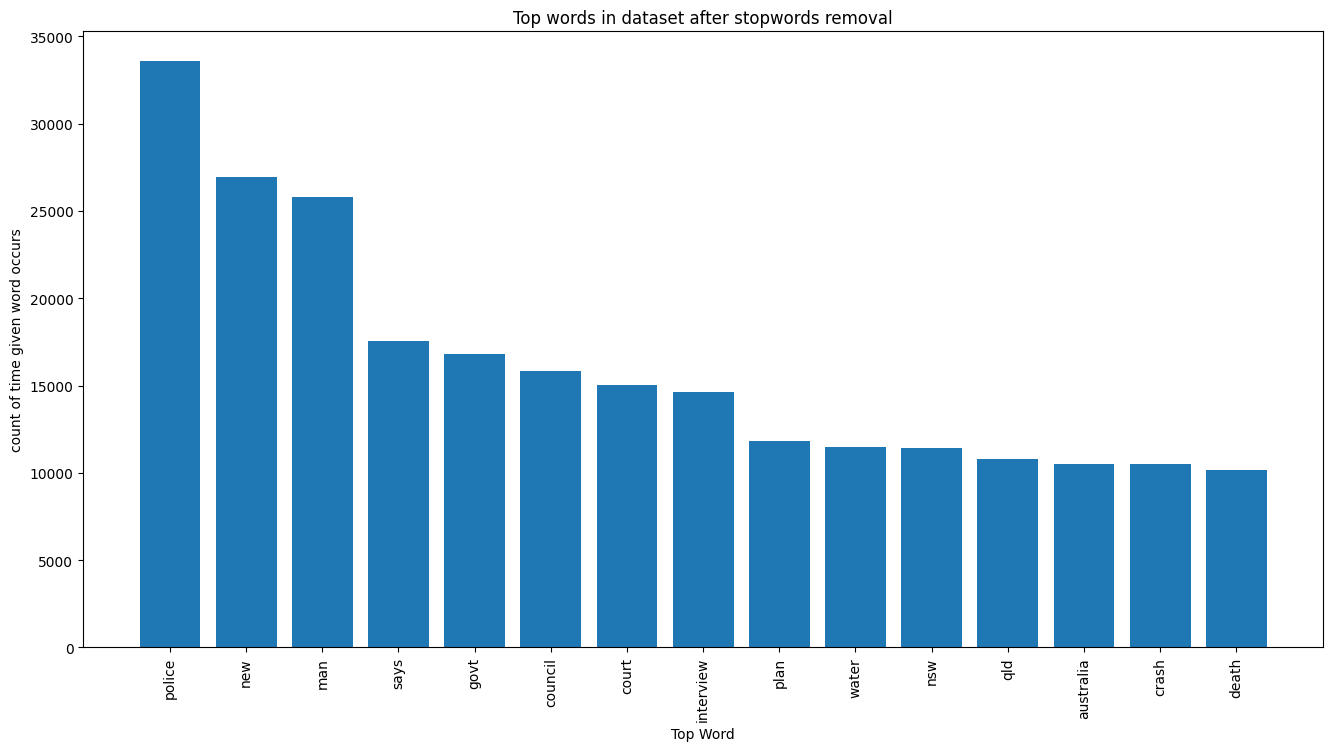The top words are 'police', 'man', 'govt' etc. These words, at some high level, refer to various topics to which the respective news can belong. For example, 'police' is a word which can be related to law and order, crime investigation, etc. but has a very minute chance of being connected to finance.

#### Daily count of news

Previously we quoted that around 200 news were witnessed by ABC daily. Let's verify the same. A plot of the daily news count corresponding to its date will serve the purpose; hence the same is shown below.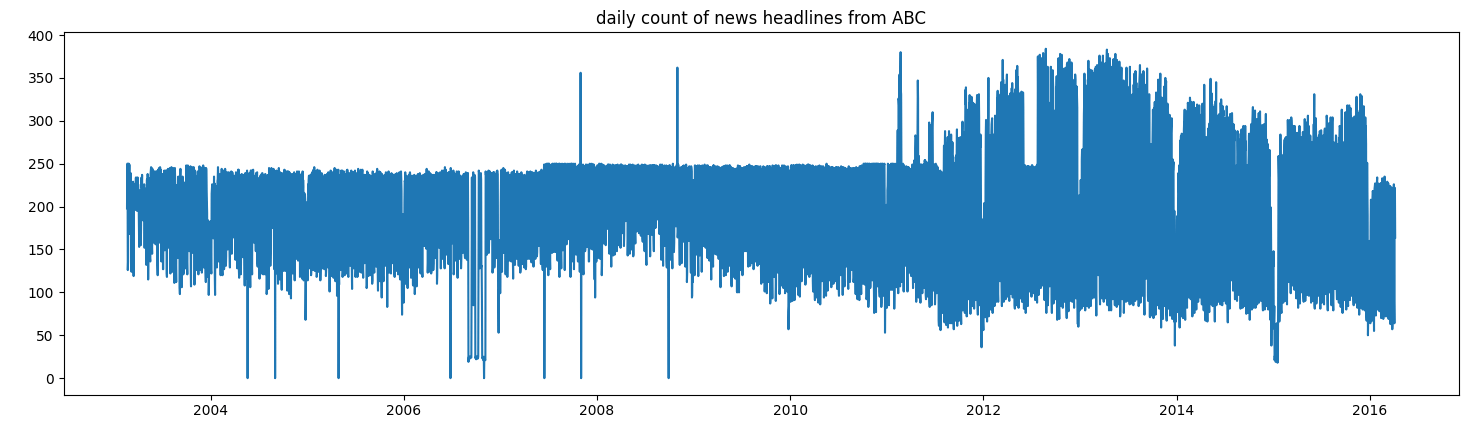We can visually infer from the plot above that the 200 news count is the average news count over a long period of around 19 years of data. Hence, we can confidently verify that approximately 200 news were published on the ABC website daily.

#### Number of words per headline

The number of words per headline is important, as both LDA and LSA depend on words to infer the topic. Extremely low or high word counts per heading will not make sense. Hence the need to visualize the distribution of the words per heading is necessary.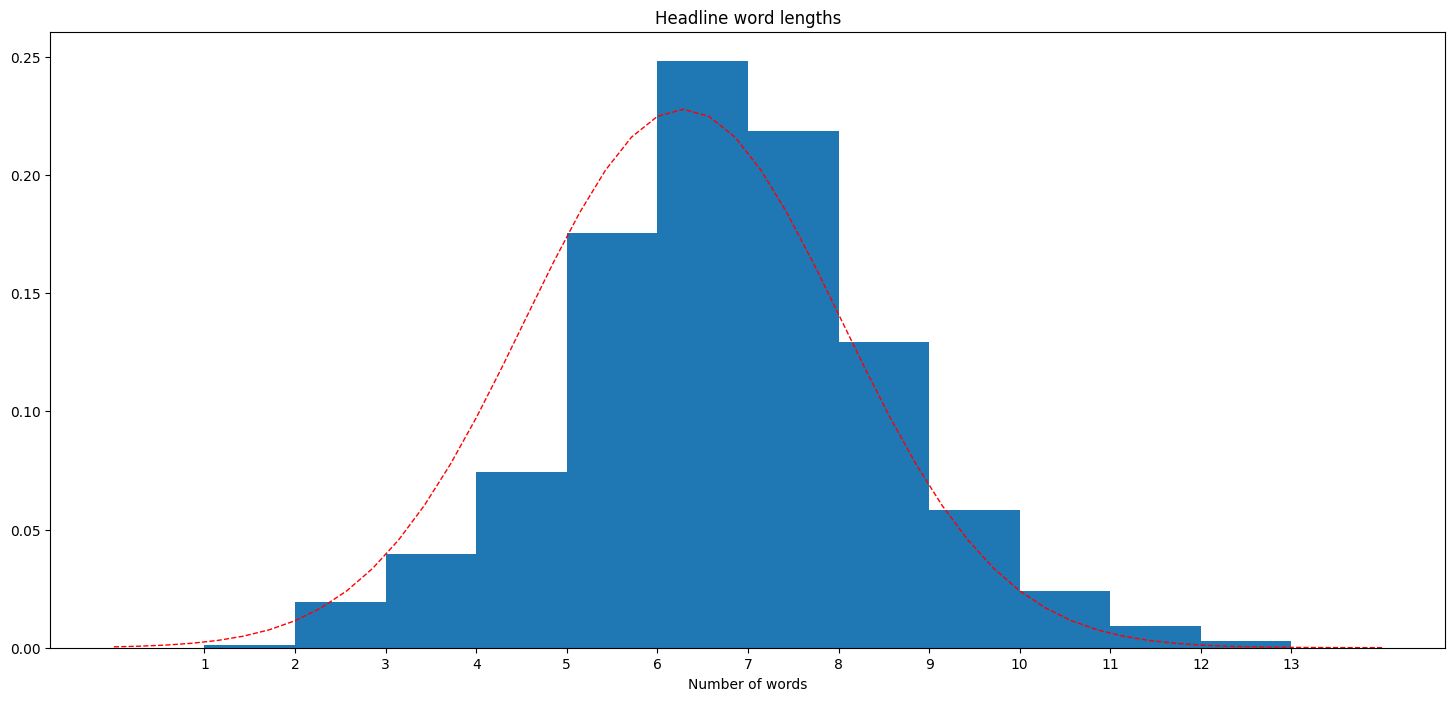The above bar graph shows us that 7 words are the most likely number of words in a news heading. Also, it is evident that extreme word counts occur very less, certifying our intuition.

The EDA has been completed. Let's build a model for LDA and LSA now.

### Steps to build the LSA and LDA model

Please note the following before the initialization of model building for these algorithms →

• Point 1: The text cannot be fed directly to the model as already discussed, and vectorizing it is the way forward. Multiple methods, such as Word2vec, TF-IDF, and count-vectorizer, are available for text vectorization. In this blog, we will be focusing only on count-vectorizer for both LDA and LSA.
• Point 2: LDA and LSA result in a probability vector corresponding to all topics. This also represents the news headings in their entirety.
The length of the probability vector per document equals the number of topics decided (10 in this Case). Overall the shape of the probability matrix comes out to be N x 10, where N is the total number of documents.
• Point 3: To visualize the clusters formed by these algorithms and to compare the cluster quality, we chose to represent each probability vector in a 2-d space using t-SNE. The better the demarcation of the topics in the formed clusters, the better the algorithm for clustering similar documents. Visualizing the distinction in the whole dataset is humanly difficult. For this reason, only 10000 randomly selected news headlines are used to build and analyze the models.

#### Document Term Matrix

As mentioned in point 1, the text needs to be vectorized using the count vectorizer method from the sklearn library. This method converts a collection of text documents to a matrix of token counts. More details can be found on the official page.

``````from sklearn.feature_extraction.text import CountVectorizer
vectorizer = CountVectorizer()
sample_document_term_matrix = vectorizer.fit_transform(small_text_sample)

## Output
Headline before vectorization: council considers hospital development plan
Headline after vectorization --> (Index of occurance in the Matrix), token count
(0, 2665) 1
(0, 2521) 1
(0, 5258) 1
(0, 3153) 1
(0, 8042) 1``````

Please note that the vectorized text is present in the form of a sparse matrix ( a lot of zeros will be present) due to the large size of the unique vocabulary. Each element in the matrix represents the count corresponding to some token. The dataset smalltextsample is a mini corpus of our main dataset formed by randomly selecting 10000 news headlines.

#### Building LSA model

We have already discussed the theoretical functioning of LSA at the start of this blog. Now let's build the LSA model using the sklearn library of Python. The input to this step is the document matrix constructed in the previous section.

``````from sklearn.decomposition import TruncatedSVD
# Initialsing the model
lsaModel = TruncatedSVD(n_components=10)
# Fit the model with dataset
lsa_matrix = lsaModel.fit_transform(sample_document_term_matrix)``````

Truncated SVD is a sklearn method used for dimensionality reduction, and unlike PCA, it works very well with sparse matrices. Hence, it works well on term-count/TF-IDF matrices as returned by the vectorizers in `sklearn.feature_extraction.text .`This method is known as LSA, which is precisely what we are doing here.

In the code snippet above, n_components refers to the number of topics in which text can be classified and is already pre-defined by us.

lsa_matrix, as mentioned, is of length 10 for each document. It outputs a probability of the documents belonging to each topic, and selecting the topic with the maximum probability from the probability vector gives us the predicted topic for that document.

The probability vectors are also an excellent topic-wise representation of the real data present in the documents. To visualize the clusters formed by LSA and to compare the cluster quality, we chose to represent each probability vector in a 2-d space using t-SNE. t-SNE is an unsupervised dimensionality reduction technique used for exploring high-dimensional data. More about this can be read in the t-SNE blog.

``````from sklearn.manifold import TSNE
tsne_lsa_model = TSNE(n_components=2, perplexity=50, learning_rate=100,
n_iter=2000, verbose=1, random_state=0, angle=0.75)
tsne_lsa_vectors = tsne_lsa_model.fit_transform(lsa_topic_matrix)``````

Let's see what each component signifies in the above t-SNE algorithm →

• n_components: Dimension of the output or embedded space.
• perplexity: It determines the number of nearest neighbours considered while computing the t-SNE algorithm. This number cannot exceed the number of samples.
• learning rate: This value has to be kept at an optimum and cannot be too high or too low. Otherwise, unexpected behaviour can be portrayed by the data.
• n_iter: Maximum iterations allowed for the algorithm to conclude. It can even stop early in case optimization is achieved.
• random_state: The value passed here determines the behaviour of the random number generator. Each time, Passing different values results in a different random initialization, affecting the local minima.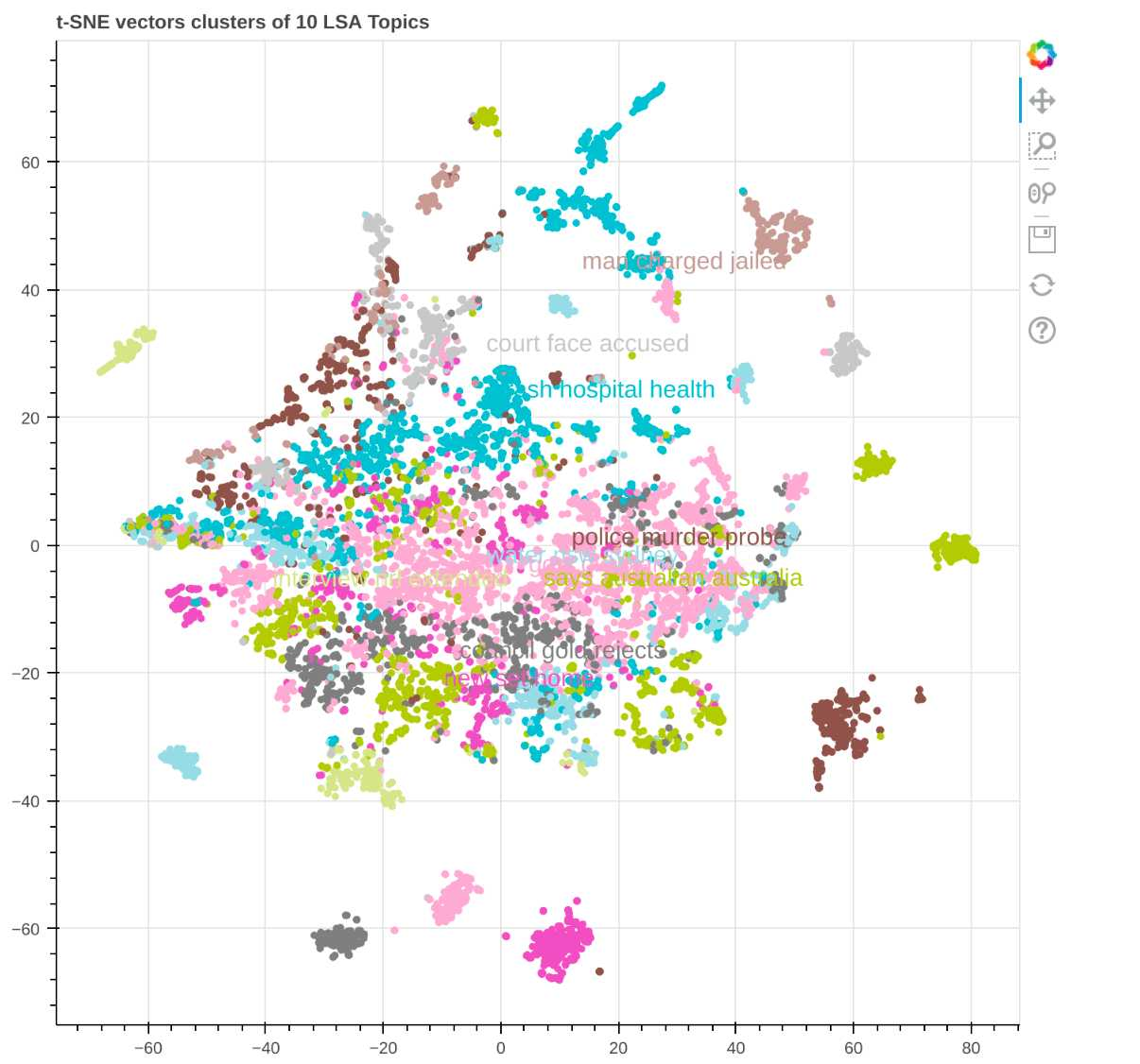Each colour in the graph above represents a topic. It is visually evident that the demarcations are not clear.
Let's now use LDA to visualize and compare its results with LSA.

#### Building LDA model

``````from sklearn.decomposition import LatentDirichletAllocation
lda_model = LatentDirichletAllocation(n_components=10, learning_method='online',
random_state=0, verbose=0)
lda_topic_matrix = lda_model.fit_transform(small_document_term_matrix)``````

We have discussed the theoretical functioning of LDA at the start of this blog. Now let's build the LDA model using the sklearn library of Python. The input to this step is the document matrix constructed in the previous section.

Let's understand the various components utilized here →

• n_components: It refers to the number of topics in which text can be classified and is already pre-defined by us.
• random_state: The value passed here determines the behaviour of the random number generator. Each time, Passing different values results in a different random initialization, affecting the local minima.

Over here, a t-SNE vector cluster is plotted on the graph for the obtained ldamatrix in the same way as we plotted before for the lsamatrix.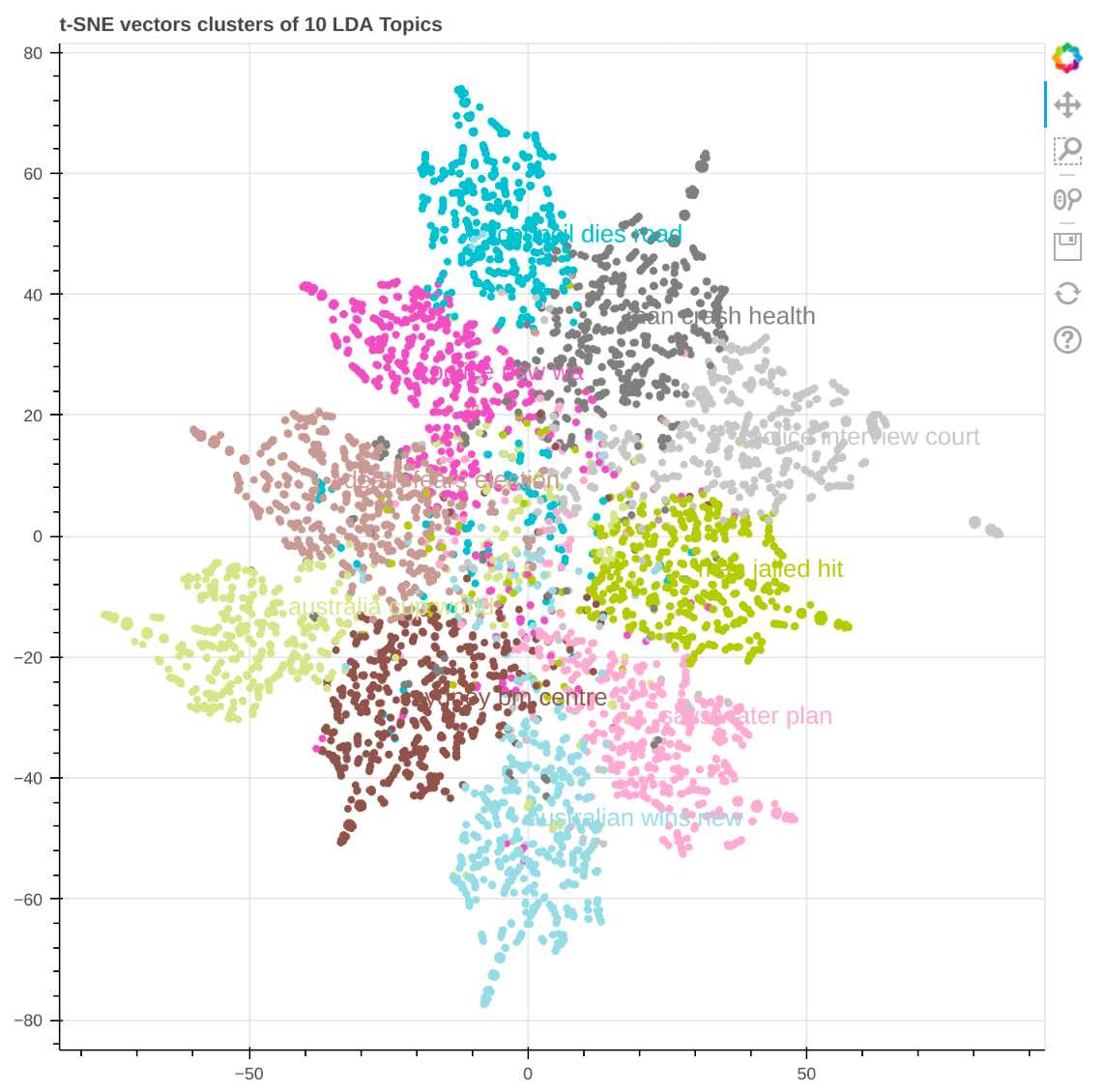Here it is visible that the topics are distinguishable and well-demarcated, unlike the haphazard demarcation in LSA, thus proving that LDA performs better than LSA for topic modelling.

Let's also construct a PCA diagram for LDA to analyze the results and compare them with those obtained using t-SNE. PCA stands for Principal Component Analysis and is a prevalent method to analyze data with high dimensional features. The code is shown below.

``````from sklearn.decomposition import PCA
pca = PCA(n_components = 2)
pca.fit(lda_topic_matrix)
x_pca_lda = pca.transform(lda_topic_matrix)``````

The PCA cluster that we received for the obtained ldatopicmatrix is shown below.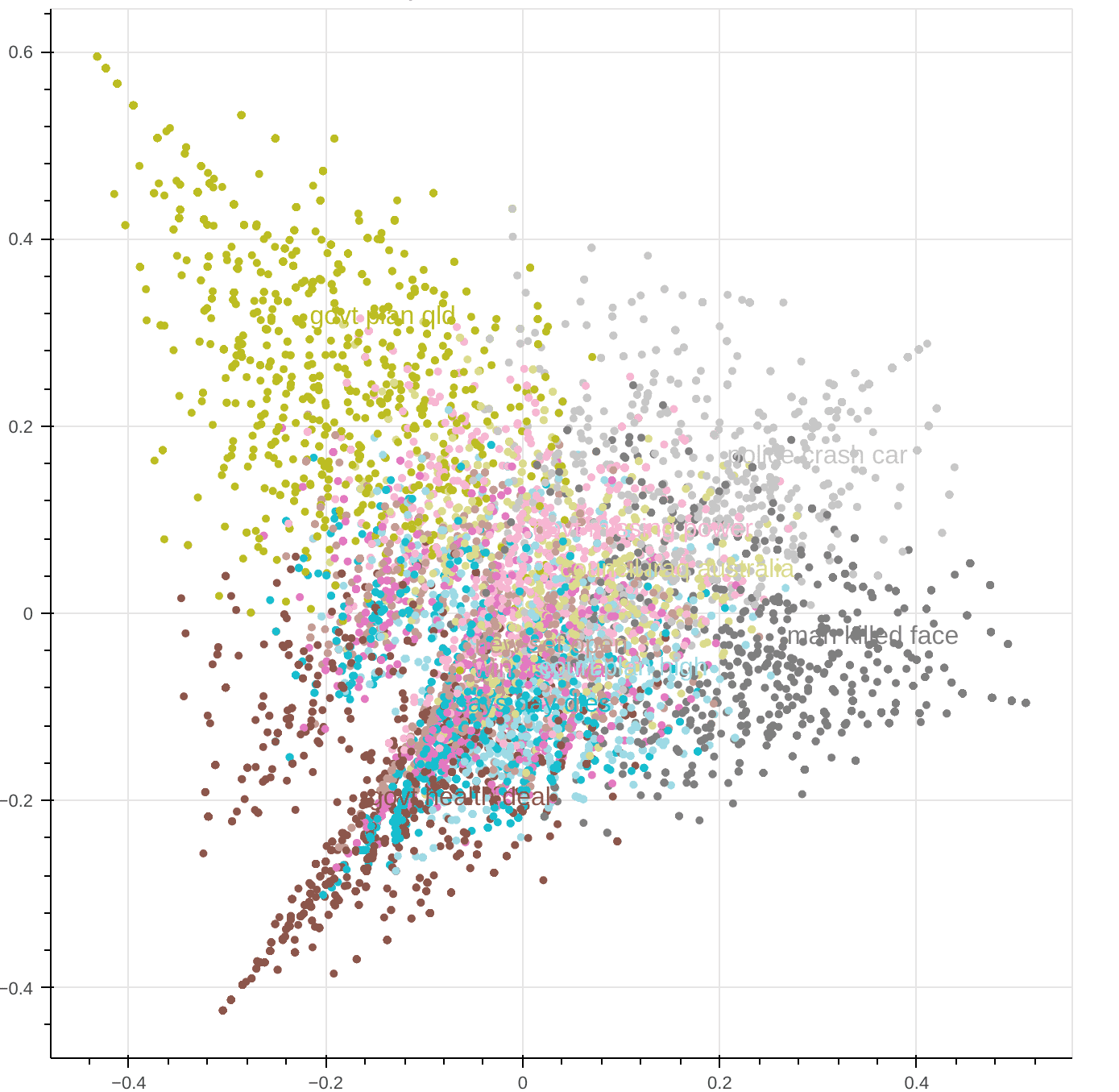It is visible from the PCA diagram above that the demarcation between each category is less distinguishable when compared to the one received in the t-SNE diagram.

This can be attributed to majorly 2 factors which are →

• PCA is a linear algorithm, while t-SNE is a non-linear algorithm.
When similarity needs to be observed, linear methods like scaling and projection might fail compared to non-linear methods.
• t-SNE is a more complicated algorithm and therefore involves more processing than PCA. At the same time, it must be remembered that a complex algorithm is not always better, but logically they tend to outperform simple ones in many cases.

More details about the above differences can be read in this blog.

### Industry use-case study

#### Aviso.AI

This company helps its customer's sales representatives to increase their chances of success in getting a deal by analyzing various risks involved related to revenue and analyzing the call between the sales representatives and the buyer. This part of analyzing the call between the sales representatives and the buyer falls under CI (Conversational Intelligence)

Here topic modelling plays a vital role. Consider a call between an Aviso sales rep and a buyer P1. A higher management person wants to review the call and only wants to know what was discussed on a particular topic, such as cost. Then this comes very handy as they can directly check all the data under the topic "cost" and quickly get to know the information.

### Interview Questions

• What is topic modelling?
• What is the need for topic modelling?
• What is LDA?
• What is LSA?
• How is SVD different from PCA

### Conclusion

Considering the boom in daily data, Topic modelling has become a vital part of today's NLP industry to select relevant and meaningful insights from the raw data. Most of these datasets are unlabelled and require unsupervised learning methods to assign topics. In this blog, we have developed a topic model using two unsupervised learning algorithms: LSA and LDA. These algorithms were discussed in detail, implemented in Python on a real dataset, followed by comparing their performance. We hope you enjoyed the article.

☆ 16-Week Live DSA Course
☆ 10-Week Live DSA Course